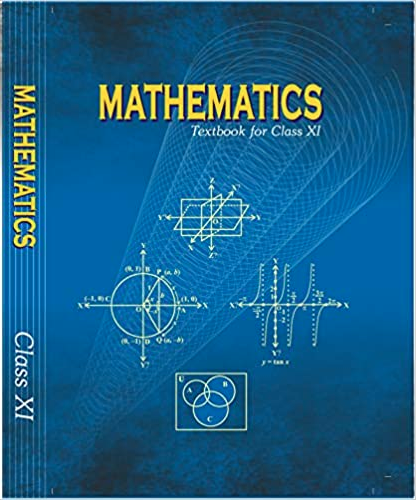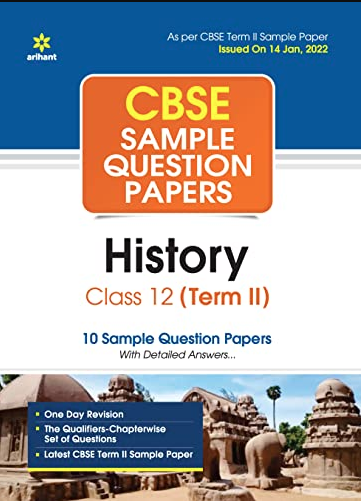# How to Prepare for MCA Exams

MCA stands for Masters of Computer Applications. This is a postgraduate degree in India, in the field of computer applications. Students opting for MCA should have Mathematics as their subjects in either 10+2 or in graduation. MCA entrance examination is held for the students seeking admission to the best national institutes as per the merit.

## Exam Preparation Tips for Masters of Computer Applications

Tip #1
Prepare well for the exam. Start preparing as soon as you plan to pursue MCA. It will be beneficial for you to be familiar with the exam.

Tip #2
One should be aware of the exam pattern. There are three sections in the paper. So the candidates should give equal time to each section in order to score well.

Tip #3
The first section tests your analytical ability. One can practice such questions from the last few years' question papers. Also some good practice books available in the market. Practicing these questions will put the candidate in a habit of answering such questions and help sharpen the mind.

Tip #4
The second section consists of the mathematical ability questions for which the candidates should thoroughly practice mental mathematics questions.

Tip #5
The third section evaluates the candidate on communication ability. This again should be well practiced. These questions may seem easy but actually are difficult to attempt and require a lot of practice and training to get the accurate answer because they are highly confusing.

Tip #6
One can start the preparation of MCA entrance in the final year of graduation. If a good amount of time, 5-6 hrs is put in, each day then 5-6 months will be more than enough for the total preparation.

Tip #7
Since this exam has an unusual pattern, one may take many mock tests for practice to get the complete idea of the exam.

Tip #8
Also, candidates must keep in mind that the questions are pretty confusing so one should take care of the time. Time management is one key factor that is responsible for success.

Tip #9
Candidates should do enough research to find out the best colleges and universities and prepare according to the particular exam particular. MCA entrance for different universities may differ.

Tip #10
One may join a preparation institute but preparation for MCA can be done by your own if given proper guidance and dedication.

Last Updated on : January 19, 2016Chapter 5 - Complex Numbers and Quadratic Equations Questions and Answers: NCERT Solutions for Class 11 MathsExercise 5.1 1. Express the given complex number in the form a+ib:(5i)(−35i)a+ib:(5i)(−35i) And evaluate Ans: Evaluate the complex number (5i)(−35i)=−5×35×i×i(5i)(−35i)=−5×35×i×i (5i)(−35i)=−3i2⋯[i2=−1](5i)(−35i)=−3i2⋯[i2=−1] (5i)(−35i)=3(5i)(−35i)=3 2. Express the given complex number in the… Read More...Chapter 4 - Principle of Mathematical Questions and Answers: NCERT Solutions for Class 11 Maths( Exercise 4.1) 1. Prove that following by using the principle of mathematical induction for all n∈Nn∈N: 1+3+32+.....+3n-1=(3n-1)21+3+32+.....+3n-1=(3n-1)2 Ans: Let us denote the given equality by P(n),P(n), i.e., P(n):1+3+32+.....+3n-1=(3n-1)2P(n):1+3+32+.....+3n-1=(3n-1)2 For… Read More...Mention any two places related to the Revolt of 1857.Mention any two places related to the Revolt of 1857. Answer. Mention any two places related to the Revolt of 1857. Jhansi, Delhi, Calcutta, Awadh, Kanpur, Meerut Read More...